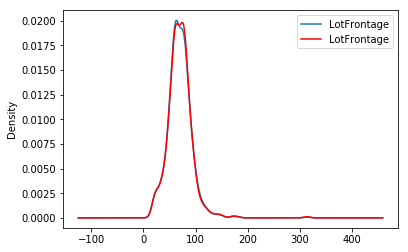# RandomSampleImputer#

The `RandomSampleImputer()` replaces missing data with a random sample extracted from the variable. It works with both numerical and categorical variables. A list of variables can be indicated, or the imputer will automatically select all variables in the train set.

Note

The random samples used to replace missing values may vary from execution to execution. This may affect the results of your work. Thus, it is advisable to set a seed.

## Setting the seed#

There are 2 ways in which the seed can be set in the `RandomSampleImputer()`:

If `seed = 'general'` then the random_state can be either `None` or an integer. The `random_state` then provides the seed to use in the imputation. All observations will be imputed in one go with a single seed. This is equivalent to `pandas.sample(n, random_state=seed)` where `n` is the number of observations with missing data and `seed` is the number you entered in the `random_state`.

If `seed = 'observation'`, then the random_state should be a variable name or a list of variable names. The seed will be calculated observation per observation, either by adding or multiplying the values of the variables indicated in the `random_state`. Then, a value will be extracted from the train set using that seed and used to replace the NAN in that particular observation. This is the equivalent of `pandas.sample(1, random_state=var1+var2)` if the `seeding_method` is set to `add` or `pandas.sample(1, random_state=var1*var2)` if the `seeding_method` is set to `multiply`.

For example, if the observation shows variables color: np.nan, height: 152, weight:52, and we set the imputer as:

```RandomSampleImputer(random_state=['height', 'weight'],
seed='observation',
```

the np.nan in the variable colour will be replaced using pandas sample as follows:

```observation.sample(1, random_state=int(152+52))
```

For more details on why this functionality is important refer to the course Feature Engineering for Machine Learning.

Note, if the variables indicated in the `random_state` list are not numerical the imputer will return an error. In addition, the variables indicated as seed should not contain missing values themselves.

## Important for GDPR#

This estimator stores a copy of the training set when the `fit()` method is called. Therefore, the object can become quite heavy. Also, it may not be GDPR compliant if your training data set contains Personal Information. Please check if this behaviour is allowed within your organisation.

Below a code example using the House Prices Dataset (more details about the dataset here).

First, let’s load the data and separate it into train and test:

```import numpy as np
import pandas as pd
import matplotlib.pyplot as plt
from sklearn.model_selection import train_test_split

from feature_engine.imputation import RandomSampleImputer

# Separate into train and test sets
X_train, X_test, y_train, y_test = train_test_split(
data.drop(['Id', 'SalePrice'], axis=1),
data['SalePrice'],
test_size=0.3,
random_state=0
)
```

In this example, we sample values at random, observation per observation, using as seed the value of the variable ‘MSSubClass’ plus the value of the variable ‘YrSold’. Note that this value might be different for each observation.

The `RandomSampleImputer()` will impute all variables in the data, as we left the default value of the parameter `variables` to `None`.

```# set up the imputer
imputer = RandomSampleImputer(
random_state=['MSSubClass', 'YrSold'],
seed='observation',
)

# fit the imputer
imputer.fit(X_train)
```

With `fit()` the imputer stored a copy of the X_train. And with transform, it will extract values at random from this X_train to replace NA in the datasets indicated in the `transform()` methods.

```# transform the data
train_t = imputer.transform(X_train)
test_t = imputer.transform(X_test)
```

The beauty of the random sampler is that it preserves the original variable distribution:

```fig = plt.figure()
X_train['LotFrontage'].plot(kind='kde', ax=ax)
train_t['LotFrontage'].plot(kind='kde', ax=ax, color='red')
lines, labels = ax.get_legend_handles_labels()
ax.legend(lines, labels, loc='best')
```### More details#

In the following Jupyter notebook you will find more details on the functionality of the `RandomSampleImputer()`, including how to set the different types of seeds.

All Feature-engine notebooks can be found in a dedicated repository.

You will also find a lot of information on why the seed matters in this notebook:

And finally, there is also a lot of information about this and other imputation techniques in this online course: